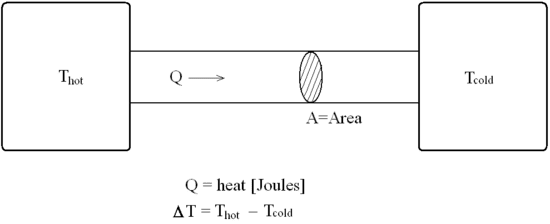# A little guidance with thermal conductivity please

• earlofwessex

#### earlofwessex

a little guidance with thermal conductivity please...

## Homework Statement

there isn't really a problem statement, as its a lab write up I'm doing.
we had two blocks of the same metal, one at room temp and one at a fixed temp of almost 50 degrees.

a Rod of different metal was put between the metal blaocks and the temperature measured. I'm looking to calculate the thermal conductivity of the rod. but the problem is that I'm out by a factor of almost 1000.

## Homework Equations

dE/dt = M C dTheta/dt.
(change in energy = Mass x SpecificHeatCapacity x Change in Temp)

Phi = Lambda A dTheta/dX
(heat energy (W) = thermal conductivity x Area x Temperature Gradient)

## The Attempt at a Solution

ok, so we're given an electric heater which cuts off at about 41 degrees, (heating up the block without attatched rod et.c gives Specific heat capacity from W=IV=M C dtheta/dt)

I'm getting 630 j/kg/K, where as its probably alluminium at 900ish. that's fine, heat lost to surroundings and so on. (but would it be better to use the official value or the calculated value for the next bit?)

so examining the cold block now that the system is set up, we can get dE/dt.
this has to be energy flowing through the rod right? so
M C dtheta/dt=Lambda A dTheta/dx

M = mass of the cold block
C = SHC of the coldd block
dTheta/dt of the cold block
Lambda of the rod
A = cross sectional area of the rod.
dTheta/dx = temperature gradient of the rod.

except that... dTheta/dx changes with time (as the cold block heats up).

so the time derivative of both sides...
dW/dt = Lambda A (dTheta/dx)/dt

where dW/dt is the change in watts over time for the block of alluminium.

this equation is giving me a value for lambda of about 1 watt per metre per kelvin. but the rod is definitely metal, and looks like copper, so the result should be up to 380. what am i doing wrong? is it better to use the published value for Specific heat capacity of alluminium? that still wouldn't get me any closer...

thanks

Bump...

I can post some figures if it would help?

this is a diagram of the setup roughly...